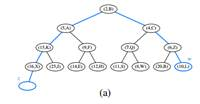### Create an Account

Home / Questions / Given a heap H and a key k give an algorithm to compute all the entries in H having a key ...

# Given a heap H and a key k give an algorithm to compute all the entries in H having a key less than or equal to k For example given the heap of Figure 912a and query k 7 the algorithm should

Given a heap H and a key k, give an algorithm to compute all the entries in H having a key less than or equal to k. For example, given the heap of Figure 9.12a and query k = 7, the algorithm should report the entries with keys 2, 4, 5, 6, and 7 (but not necessarily in this order). Your algorithm should run in time proportional to the number of entries returned, and should not modify the heap.

Figure 9.12aJun 13 2020 View more View LessSubscribe To Get Solution